# Subcarrier Multiplexing (SCM)

In some LAN and MAN applications the bit rate of each channel is relatively low but the number of channels can become quite large. An example is provided by common antenna (cable) television (CATV) networks. The basic concept behind subcarrier multiplexing (SCM) is borrowed from microwave technology, which employs multiple microwave carriers for transmitting multiple CATV channels (electrical FDM) over coaxial cables or free space. The total bandwidth is limited to well below 1 GHz when coaxial cables are sued to transmit a multichannel microwave signal. However, if the microwave signal is transmitted optically by using optical fibers, the signal bandwidth can easily exceed 10 GHz for a single optical carrier. Such a scheme is referred to as SCM, since multiplexing is done by using microwave subcarriers rather than the optical carrier. It has been used commercially by the CATV industry since 1992 and can be combined with TDM or WDM. A combination of SCM and WDM can realize bandwidths in excess of 1 THz. The SCM technique essentially sends a radio or microwave signal over optical fibers and is also referred to as radio over fibers.

The following figure shows schematically a SCM lightwave system designed with a single optical carrier. The main advantage of SCM is the flexibility and the upgradability offered by it in the design of broadband networks. On can use analog or digital modulation, or a combination of the two, to transmit multiple voice, data, and video signals to a large number of users. Each user can be served by a single subcarrier, or the multichannel signal can be distributed to all users as done commonly by the CATV industry. The SCM technique has been studied extensively because of its wide-ranging practical applications. In this section we describe both the analog and digital SCM systems with emphasis on their design and performance.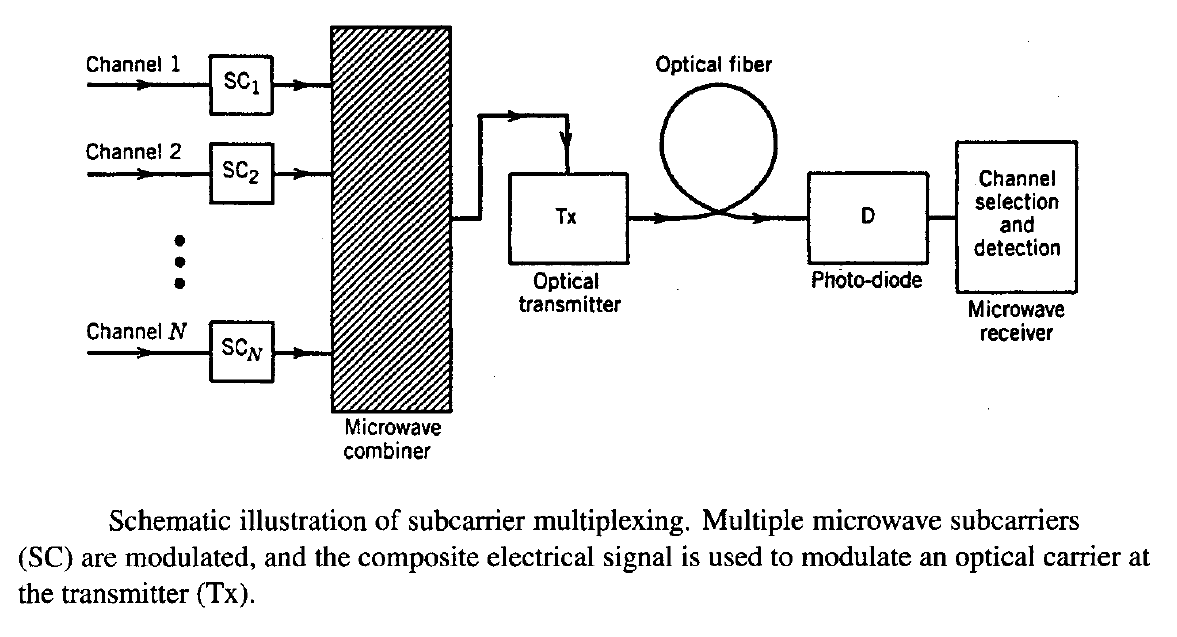#### 1. Analog and Digital SCM Systems

In fiber optic communication networks we focus mostly on digital modulation techniques as they are employed almost universally for lightwave systems. An exception occurs in the case of SCM systems designed for video distribution. Until 2000, most CATV networks distributed television channels by using analog techniques based on frequency modulation (FM) or amplitude modulation with vestigial sideband (AM-VSB) formats. As the wave form of an analog signal must be preserved during transmission, analog SCM systems require a high SNR at the receiver and impose strict linearity requirements on the optical source and the communication channel.

In analog SCM lightwave systems, each microwave subcarrier is modulated using an analog format, and the output of all subcarriers is summed using a microwave power combiner (see the figure above). The composite signal is used to modulate the intensity of a semiconductor laser directly by adding it to the bias current. The transmitted power can be written as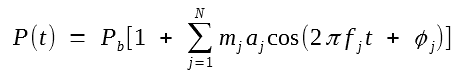where Pb is the output power at the bias level and mj, aj, fj, and φj are, respectively, the modulation index, amplitude, frequency, and phase associated with the jth microwave subcarrier; aj, fj, or φj is modulated to impose the signal depending on whether AM, FM, or phase modulation (PM) is used.

The power at the receiver would also be in the form of the above equation if the communication channel were perfectly linear. In practice, the analog signal is distorted during its transmission through the fiber link. The distortion is referred to as intermodulation distortion (IMD) and is similar in nature to the Four-Wave Mixing (FWM) distortion discussed in previous tutorials. Any nonlinearity in the response of the semiconductor laser used inside the optical transmitter or in the propagation characteristics of fibers generates new frequencies of the form fi + fj, and fi + fj ± fk, some of which lie within the transmission bandwidth and distort the analog signal. The new frequencies are referred to as the intermodulation products (IMP). These are further subdivided as two-tone IMPs and triple-beat IMPs, depending on whether two frequencies coincide or all three frequencies are distinct. The triple-beat IMPs tend to be a major source of distortion because of their large number. An N-channel SCM system generates N(N - 1)(N - 2)/2 triple-beat terms compared with N(N - 1) two-tone terms. The second-order IMD must also be considered if subcarriers occupy a large bandwidth.

IMD has its origin in several distinct nonlinear mechanisms. The dynamic response of semiconductor lasers is governed by the rate equations, which are intrinsically nonlinear. The solution of these equations provides expressions for the second- and third-order IMPs originating from this intrinsic nonlinearity. Their contribution is largest whenever the IMP frequency falls near the relaxation-oscillation frequency. A second source of IMD is the nonlinearity of the power-current curve. The magnitude of resulting IMPs can be calculated by expanding the output power in a Taylor series around the bias power. Several other mechanisms, such as fiber dispersion, frequency chirp, and mode-partition noise can cause IMD, and their impact on the SCM systems has been studied extensively.

The IMD-induced degradation of the system performance depends on the interchannel interference created by IMPs. Depending on the channel spacing among microwave subcarriers, some of the IMPs fall within the bandwidth of a specific channel and affect the signal recovery. It is common to introduce composite second-order (CSO) and composite triple-beat (CTB) distortion by adding the power for all IMPs that fall within the passband of a specific channel. The CSO and CTB distortion values are normalized to the carrier power of that channel and expressed in dBc units, where the "c" in dBc denotes normalization with respect to the carrier power. Typically, CSO and CTB distortion values should be below -60 dBc for negligible impact on the system performance; both of them increase rapidly with an increase in the modulation index.

System performance depends on the SNR associated with the demodulated signal. In the case of SCM systems, the carrier-to-noise ratio (CNR) is often used in place of SNR. The CNR is defined as the ratio of root mean square (RMS) carrier power to RMS noise power at the receiver and can be written aswhere m is the modulation index, R is the detector responsivity,is the average received optical power, and σs, σT, σI, and σIMD are the RMS values of the noise currents associated with the shot noise, thermal noise, intensity noise, and IMD, respectively. If we assume that the relative intensity noise (RIN) of the laser is nearly uniform within the receiver bandwidth,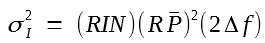The RMS value of σIMD depends on the CSO and CTB distortion values.

The CNR requirements of SCM systems depend on the modulation format. In the case of AM-VSB format, the CNR should typically exceed 50 dB for satisfactory performance. Such large values can be realized only by increasing the received optical powerto a relatively large value (> 0.1 mW). This requirement has two effects. First, the power budget of AM-analog SCM systems is extremely limited unless the transmitter power is increased above 10 mW. Second, the intensity-noise contribution to the receiver noise dominates the system performance as σI2 increases quadratically with. In fact, the CNR becomes independent of the received optical power when σI dominates. From the two equations above the limited value of CNR is given by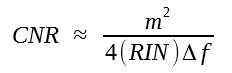As an example, the RIN of the transmitter lsaer should be below -150 dB/Hz to realize a CNR of 50 dB if m = 0.1 and Δf = 50 MHz are used as the representative values. Larger values of RIN can be tolerated only by increasing the modulation index m or by decreasing the receiver bandwidth. Indeed, DFB lasers with low values of the RIN were developed during the 1990s for CATV applications. In general, the DFB laser is biased high above threshold to provide a bias power Pb in excess of 5 mW because its RIN decreases as Pb-3. High values of the bias power also permit an increase in the modulation index m.

The intensity noise can become a problem even when the transmitter laser is selected with a low RIN value to provide a large CNR. The reason is that the RIN can be enhanced during signal transmission inside optical fibers. One such mechanism is related to multiple reflections between two reflecting surfaces along the fiber link. The two reflective surfaces act as an FP interferometer which converts the laser-frequency noise into intensity noise. The reflection-induced RIN depends on both the laser linewidth and the spacing between reflecting surfaces. It can be avoided by using fiber components (splices and connectors) with negligible parasitic reflections (< -40 dB) and by using lasers with a narrow linewidth (< 1 MHz). Another mechanism for the RIN enhancement is provided by the dispersive fiber itself. Because of GVD, different frequency components travel at slightly different speeds. As a result, frequency fluctuations are converted into intensity fluctuations during signal transmission. The dispersion-induced RIN depends on laser linewidth and increases quadratically with fiber length. Fiber dispersion also enhances CSO and CTB distortion for long link lengths. It becomes necessary to use a dispersion-management technique for such SCM systems.

The CNR requirement can be relaxed by changing the modulation format from AM to FM. The bandwidth of a FM subcarrier is considerably larger (30 MHz in place of 4 MHz). However, the required CNR at the receiver is much lower (about 16 dB in place of 50 dB) because of the so-called FM advantage that yields a studio-quality video signal (> 50-dB SNR) with only 16-dB CNR. As a result, the optical power needed at the receiver can be as small as 10 μm. The RIN is not much of a problem for such systems as long as the RIN value is below -135 dB/Hz. In fact, the receiver noise of FM systems is generally dominated by the thermal noise. Both the AM and FM techniques have been used successfully for analog SCM lightwave systems.

During the 1990s, the emphasis of SCM systems shifted from analog to digital modulation. The frequency-shift keying (FSK) format was used for modulating microwave subcarriers as early as 1990 but its use requires coherent detection. Moreover, a single digital video channel requires a bit rate of 100 Mb/s or more, in contrast with analog channels that occupy a bandwidth of only 6 MHz. For this reason, other modulation formats such as quadrature AM (QAM) and quadrature PSK (QPSK) have been explored. A multilevel QAM format is used often in practice with typically 64 levels. Such a signal requires a lower CNR compared with that needed for analog AM-VSB systems. The capacity of an SCM system can be increased considerably by employing hybrid techniques that mix analog and digital formats.

The hybrid SCM systems that combine the analog AM-VSB format with the digital QAM format have attracted attention because they can transmit a large number of video channels over the same fiber simultaneously. The performance of such systems is affected by the clipping noise, multiple optical reflections, and the nonlinear mechanisms such as self-phase modulation (SPM) and SBS, all of which limit the total power and the number of channels that can be multiplexed. Nevertheless, hybrid SCM systems can transport up to 80 analog and 30 digital channels using a single optical transmitter. If only QAM format is employed, the number of digital channels is limited to about 80. In a 2000 experiment, 78 channels with the 64-QAM format were transmitted over 740 km. Each channel had a bit rate of 30 Mb/s, resulting in a total capacity of 2.34 Gb/s. Such a SCM system can transport up to 500 compressed video channels. Further increase in the system capacity can be realized by combining the SCm and WDM techniques, a topic discussed next.

#### 2. Multiwavelength SCM Systems

The combination of WDM and SCM provides the potential of designing broadband passive optical networks capable of providing integrated devices (video, data, etc.) to a large number of subscribers. In this scheme, shown in the figure below, multiple optical carriers are launched into the same optical fiber through the WDM technique. Each optical carrier carries multiple SCM channels using several microwave subcarriers. One can mix analog and digital signals using different subcarriers or different optical carriers. Such networks are extremely flexible and easy to upgrade as the demand grows.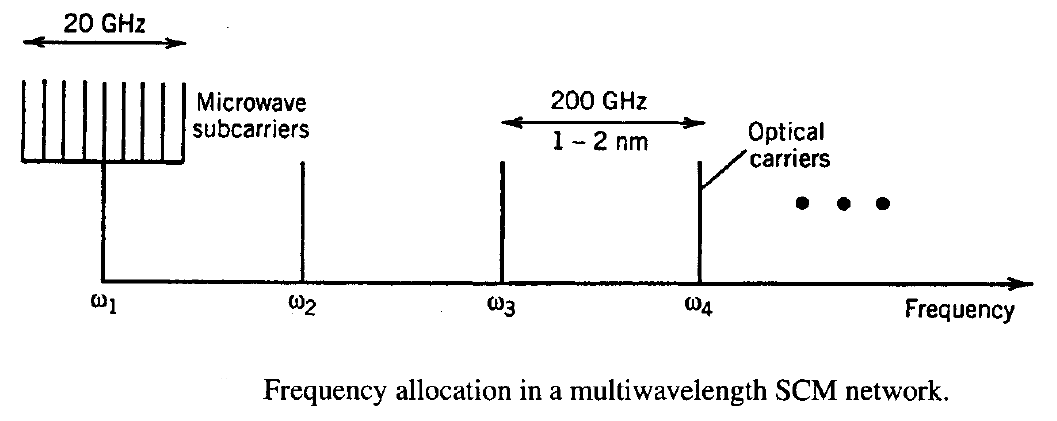As early as 1990, 16 DFB lasers with a wavelength spacing of 2 nm in the 1.55-μm region were modulated with 100 analog video channels and six 622-Mb/s digital channels. Video channels were multiplexed using the SCM technique such that one DFB laser carried 10 SCM channels over the bandwidth 300-700 MHz. The potential of such WDM systems was demonstrated in a 2000 experiment in which a broadcast-and-select network was capable of delivering 10,000 channels, each operating at 20 Gb/s. The network used 32 wavelengths (on the ITU grid) each of which could carry 310 microwave subcarriers by modulating at a composite bit rate of 20 Gb/s. In a 2002 experiment, 8 WDM channels were transmitted over 800 km of fiber. Each optical channel delivered 1.04-Gb/s payload using 35 subcarriers, each carrying a 32.2-Mb/s signal in the 256-QAM format.

The limiting factor for multiwavelength SCM networks is interchannel crosstalk resulting from both the linear and nonlinear processes. The nonlinear effects that produce interchannel crosstalk are SRS and XPM, both of which have been analyzed. The figure below shows the crosstalk measured in a two-channel experiment together with the theoretical prediction of the SRS- and XPM-induced crosstalk levels. One channel is modulated and carries the actual signal while the other operates continuously (CW) but its power is low enough that it acts as a probe.The wavelength difference λmod - λCW is ±8.5 nm in the two cases. The probe power varies with time because of SRS and XPM, and the crosstalk is defined as the ratio of radio-frequency (RF) powers in the two channels. The XPM-induced crosstalk increases and the Raman-induced crosstalk decreases with the modulation frequency but each has the same magnitude in the two cases. The two crosstalks add up in phase only when λmod < λCW, resulting in a larger value of the total crosstalk in that case. The asymmetry is due to SRS and depends on whether the CW probe channels is being depleted or is being amplified by the other channel.

The linear crosstalk results from the phenomenon of optical beat interference. It occurs when two or more users transmit simultaneously on the same optical channel suing different subcarrier frequencies.  As the optical carrier frequencies are then slightly different, their beating produces a beat note in the photocurrent. If the beat-note frequency overlaps an active subcarrier channel, an interference signal would limit the detection process in a way similar to IMD. Statistical models have been used to estimate the probability of channel outage because of optical beat interference.

Multiwavelength SCM systems are quite useful for LAN and MAN applications. They can provide multiple services (telephone, analog and digital TV channels, computer data, etc.) with only one optical transmitter and one optical receiver per user because different services can use different microwave subcarriers. This approach lowers the cost of terminal equipment in access networks. Different services can be offered without requiring synchronization, and microwave subcarriers can be processed using commercial electronic components. Each user is assigned a unique wavelength for transmitting multiple SCM messages but can receive multiple wavelengths. The main advantage of multiwavelength SCM is that the network can serve NM users, where N is the number of optical wavelengths and M is the number of microwave carriers by using only N distinct transmitter wavelengths. The optical wavelengths can be relatively far apart (coarse WDM) for reducing the cost of the terminal equipment. In another approach, the hybrid fiber/coaxial (HFC) technology is used to provide broadband integrated services to the subscriber. Digital video transport systems operating at 10 b/s by combining the WDM and SCM techniques became available in 1996. The use of WDM and SCM for personal communication networks is quite appealing. High spectral efficiency multi-user architecture was demonstrated in 2008 capable of delivering a 1-Gb/s SCM signal per WDM channel in the 16-QAM format.

#### 3. Orthogonal Frequency-Division Multiplexing

Orthogonal frequency-division multiplexing (OFDM)  is a well-known multiplexing technique in the context of cell phones and other wireless applications. Its use for WDM systems, pursued since 2005, falls in the category of SCM systems, because OFDM, by its very nature, employs a large number of microwave subcarriers. The main difference from the SCM techniques discussed earlier in this section is the orthogonality of these subcarriers, a feature that allow their much tighter packing and thus improves the spectral efficiency considerably.

Here is an excellent explanation of OFDM on youtube.

The basic idea idea behind OFDM makes use of the discrete Fourier transform (DFT) operation. The following figure shows schematically the designs of typical OFDM transmitters and receivers.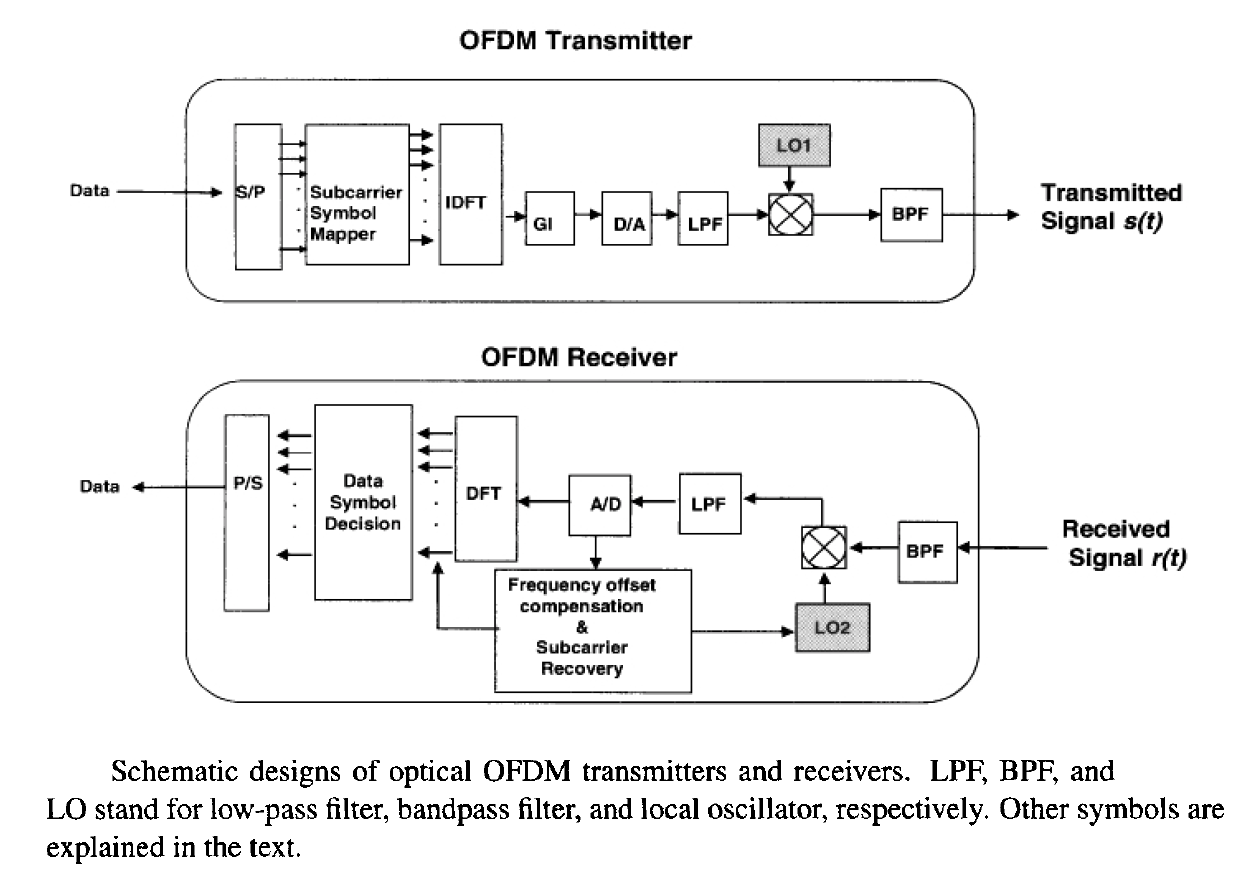As seen there, the electrical bit stream undergoes considerable digital signal processing (DSP) both at the transmitter and receiver ends. The most important part is the DFT and inverse DFT (IDFT) operations. At the transmitter end, serial data is parallelized (S/P operation) and converted into a symbol stream. The number N of parallel streams is chosen in the form of N = 2n to perform the inverse DFT operation with the help of a fast Fourier transform algorithm, the integer n being typically in the range of 6 to 10. Each parallel stream represents a microwave subcarrier after the IDFT operation. After inserting a cyclic prefix or guardband (GI operation), a digital-to-analog converter (D/A operation) is employed to obtain a composite signal containing all subcarriers. After upshifting signal frequencies by fLO using a microwave oscillator (LO1), the signal takes the formwhere sk(t) represents the kth subcarrier at frequency fLO + fk, Ts is the OFDM symbol duration, and h(t) = 1 in the interval 0 < t Ts but zero outside of it. This composite signal is used to modulate an optical carrier at a specific frequency on the ITU grid. At the receiver end, all operations are reversed to recover the original data bit stream.

To understand the origin of the orthogonality of subcarriers, first note that the DFT frequencies associated with a periodic time-dependent function (period Ts) are given by  fk = (k - 1)/Ts, where the integer k takes values from 1 to N. The subcarriers thus form a comb of uniformly spaced frequencies. The orthogonality of subcarriers follows from the relation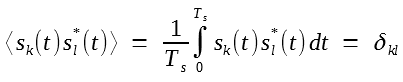where angle brackets denote averaging over the entire OFDM symbol duration. It is important to note that Ts = NTb, where Tb is the bit slot, because the input bit stream is divided into N parallel streams. Since N often exceeds 100 and can be close to 1000, Ts is much longer than Tb. In other words, the symbol rate of each subcarrier is B/N. The OFDM technique permits us to send an input bit stream in the form of N symbol streams, each on its own subcarrier. The spectra of two neighboring symbol streams overlap considerably. However, they can still be demodulated at the receiver because of the orthogonality of these subcarriers.

A major advantage of the OFDM technique is that linear distortions of the transmitted signal, including those induced by fiber dispersion, are reduced dramatically because many low-speed symbol streams are transmitted in parallel rather than one high-speed bit stream. This becomes clear by noting that, since symbol duration of each subcarrier is much longer than the bit duration, dispersive effects are less of a problem to begin with and can be removed easily at the receiver end if a cyclic prefix is added to each symbol. In this approach, the symbol duration Ts is increased by a certain amount, and this guardband at the front end is used to store a copy of the signal from the back end in a cyclic fashion. Although the increase in Ts reduces the symbol rate of each subcarrier, resulting in a net decrease of the total bit rate, the OFDM system becomes much more resistant to intersymbol interference induced by linear distortions.

The dispersion-tolerant nature of OFDM was demonstrated in a 2007 experiment, that transmitted a 8-Gb/s bit stream by using 128 subcarriers with the QPSK format. The resulting optical OFDM signal could be transmitted over 1000 km of standard telecommunication fiber (using a recirculating fiber loop) without requiring any dispersion compensation. This experiment employed two narrowband lasers (one at the transmitter and the other at the receiver) with line widths of around 20 kHz. Such narrowband lasers are needed because of a relatively low symbol rate of subcarriers and the coherent detection used for them. A transmission distance of 4160 km at a bit rate of 25.8 Gb/s was realized in another OFDM experiment in which 256 subcarriers were used. The experiment implemented a phase-noise compensation scheme by inserting a radio-frequency (RF) pilot at the transmitter. This compensation scheme is discussed later where we focus on coherent OFDM systems. Nonlinear crosstalk resulting from Four-Wave Mixing (FWM) become quite critical for OFDM systems because of a relatively small frequency spacing among subcarriers. However, with suitable modifications of the existing DSP (digital signal processing) hardware, such distortions can be reduced considerably with a combination of pre- and post-compensation at the transmitter and receiver ends.